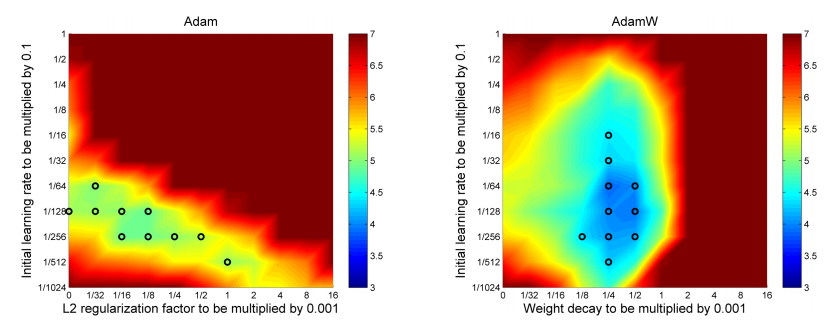# Researchers have improved the widely used deep learning optimizer Adam, decreasing the time needed for training models and improving model generalization. We made a small contribution of our own by open sourcing our PyTorch implementation of QHAdamW.

The Adam optimizer is one of the most commonly used optimizers for deep learning. When training with Adam the model usually converges a lot faster than when using regular stochastic gradient descent (SGD), and Adam often requires less tuning of the learning rate compared to SGD with momentum. Adam improves on SGD with momentum by (in addition to momentum) also computing adaptive learning rates for each parameter that is tuned. This means that when using Adam there is less need to modify the learning rate during the training than when using SGD. For a more detailed description of Adam and other optimization algorithms, see the blog post An overview of gradient descent optimization algorithms by Sebastian Ruder.

Adam is unfortunately not without flaws. In many research papers the best generalization results are achieved by SGD with momentum coupled with a well-tuned learning rate schedule. While training with Adam helps in getting fast convergence, the resulting model will often have worse generalization performance than when training with SGD with momentum. Another issue is that even though Adam has adaptive learning rates its performance improves when using a good learning rate schedule. Especially early in the training it is beneficial to use a lower learning rate to avoid divergence. This is because in the beginning the model weights are random, and thus the resulting gradients are not very reliable. A learning rate that is too large might result in the model taking too large steps and not settling in on any decent weights. When the model overcomes these initial stability issues the learning rate can be increased to speed up convergence. This process is called learning rate warm-up, and one version of it is describe in the paper Accurate, Large Minibatch SGD: Training ImageNet in 1 Hour.

The following recently published optimizers improve Adam in different ways and try to alleviate some of the issues mentioned above:

In the common weight decay implementation in the Adam optimizer the weight decay is implicitly bound to the learning rate. This means that when optimizing the learning rate you will also need to find a new optimal weight decay for each learning rate you try. The AdamW optimizer decouples the weight decay from the optimization step. This means that the weight decay and learning rate can be optimized separately, i.e. changing the learning rate does not change the optimal weight decay. The result of this fix is a substantially improved generalization performance; models trained with AdamW using an annealing learning rate schedule achieve results on image recognition tasks (e.g. CIFAR-10 and ImageNet) that match the ones achieved with SGD + momentum.As seen in this figure from the AdamW paper, the optimal weight decay in Adam is dependent on the learning rate, but in AdamW they are independent.

For a more detailed explanation on the AdamW algorithm, see Ruder's blog post Optimization for Deep Learning Highlights in 2017.

TensorFlow

## Implementations

PyTorch & TensorFlow

QHAdamW combines the weight decay decoupling from AdamW to the weight update rule changes from QHAdam, as suggested in the QHAdam paper. This is an implementation we made ourselves at IPRally. The end results we achieved with it are about the same as with AdamW, but in the early stages of the training QHAdamW performs a bit better (when using the default parameters given in the QHAdam documentation).

# LAMB

The LAMB optimizer aims to eliminate the need of the learning rate warm-up needed in the early stages of training. This is done by automatically scaling the weight update layer-wise by the ratio of the weight magnitude and the gradient magnitude for that layer. Intuitively the idea is that if the gradient magnitude is large compared to the weight magnitude then the weights for that layer are very bad, and the gradients are thus not reliable. In that case it makes sense to take small steps to avoid "jumping around" too much. On the other hand, if the magnitude of the gradients is small compared to the magnitude of the weights then it is probable that the weights are already quite good. In this case the gradient estimate is more reliable and a larger step size is warranted. In the paper LAMB is demonstrated to work well even with very large batch sizes: The full BERT model is trained in just 76 minutes (compared to 3 days in the original paper), and a ResNet-50 network can be trained on ImageNet in just a few minutes.

For a more thorough review of the LAMB algorithm, see the blog post An intuitive understanding of the LAMB optimizer written by Ben Mann.